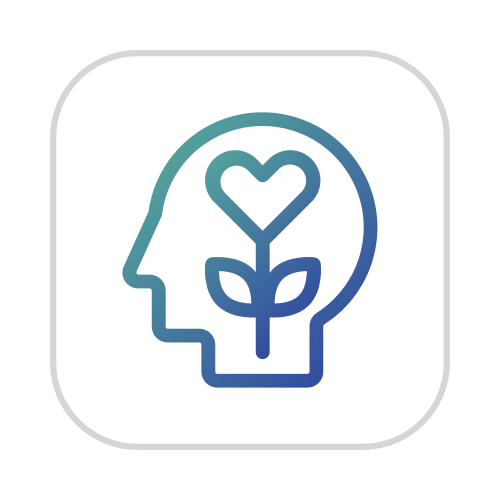# Meet the author

Scroll to read their bio 👇English BFrench BPsychologyGeography###### Michael Haese
Haese Mathematics

"I completed a Bachelor of Science at the University of Adelaide, majoring in Infection and Immunity, and Applied Mathematics. I studied laminar heat flow as part of his Honours in Applied Mathematics, and finished a PhD in high speed fluid flows in 2001. Since 2008, I have been the principal editor for Haese Mathematics."

### What motivates you to write mathematics books?

"My passion is for education as a whole, rather than just mathematics. In Australia I think it is too easy to take education for granted, because it is seen as a right but with too little appreciation for the responsibility that goes with it. But the more I travel to places where access to education is limited, the more I see children who treat it as a privilege, and the greater the difference it makes in their lives. But as far as mathematics goes, I grew up with mathematics textbooks in pieces on the kitchen table, and so I guess it continues a tradition."

### What do you aim to achieve in writing?

"I think a few things:

• I want to write to the student directly, so they can learn as much as possible from the text directly. Their book is there even when their teacher isn't.
• I therefore want to write using language which is easy to understand. Sure, mathematics has its big words, and these are important and we always use them. But the words around them should be as simple as possible, so the meaning of the terms can be properly explained to ESL (English as a Second Language) students.
• I want to make the mathematics more alive and real, not by putting it in contrived "real-world" contexts which are actually over-simplified and fake, but rather through its history and its relationship with other subjects."

### What interests you outside mathematics?

"Lots of things! Horses, show jumping and course design, alpacas, badminton, running, art, history, faith, reading, hiking, photography ...."

All mathematics content SMARTPREP features was developed by Haese Mathematics.

Haese Mathematics is a family-owned publishing company specializing in mathematics textbooks and accompanying software for schools and students since 1979.

The combination of Haese Mathematics' expertise in delivering high quality content and SMARTPREP’s passion for effective learning results in a truly unique solution for IB DP Mathematics.

Content overview

2014 2021 NewThree types of questions:Essential concepts

• “Concept” questions are designed to test a student’s understanding of concepts and terminology.
• “What is the formula for?” and “How do I use it?” are two questions a student should be able to answer confidently during revision.Skills and practice

• “Skill” questions are designed to test fundamental skills that every student should know. These questions are usually non-contextual. So, the focus stays on the skill that’s being asked for, rather than reading comprehension skills.Application and
explanation

• “Application” questions are designed to get students applying mathematical tools to real world problems and interpreting the results in context. Many of these questions will require more than one skill to answer, and may be cross-topic. Perfect for exam practice and preparation.
 SL content completion: 100% SMARTPREP IB App View content in app SMARTPREP IB Flash Cards The print option will launch in Summer 2020. Subscribe to our newsletter to stay updated.Topic 1: Number and algebraSets and Venn diagramsScientific notationSequences and seriesCounting and the binomial theoremExponents and logarithmsReasoning and proofTopic 2: FunctionsStraight linesRelations and functionsTransformations of functionsQuadraticsRational functionsExponential and logarithmic functionsUnfamiliar functionsTopic 3: Geometry and trigonometryMeasurementCoordinate geometryRight angled triangle trigonometryNon-right angled triangle trigonometryThe unit circle and radian measureTrigonometric functionsTrigonometric equations and identitiesTopic 4: StatisticsProbabilitySamplingOrganising and displaying dataDescriptive statisticsBivariate statisticsDiscrete random variablesThe binomial distributionThe normal distributionTopic 5: CalculusIntroduction to differential calculusRules of differentiationProperties of curvesApplications of differentiationIntegrationKinematics
 HL content completion: 80% SMARTPREP IB App All content finished by February 2020. New content uploaded continuously. The presented sub-topic order might be subject to change. View content in app SMARTPREP IB Flash Cards The print option will launch in Summer 2020. Subscribe to our newsletter to stay updated.Topic 1: Number and algebraSets and Venn diagramsScientific notationSequences and seriesCounting and the binomial theoremExponents and logarithmsLinear algebraReasoning and proofComplex numbersTopic 2: FunctionsStraight linesRelations and functionsTransformations of functionsQuadraticsReal polynomialsModulus equations and inequalitiesRational functionsExponential and logarithmic functionsUnfamiliar functionsTopic 3: Geometry and trigonometryMeasurementCoordinate geometryRight angled triangle trigonometryNon-right angled triangle trigonometryThe unit circle and radian measureTrigonometric functionsTrigonometric equations and identitiesVectorsTopic 4: StatisticsProbabilitySamplingOrganising and displaying dataDescriptive statisticsBivariate statisticsDiscrete random variablesThe binomial distributionContinuous random variablesThe normal distributionTopic 5: CalculusLimitsIntroduction to differential calculusRules of differentiationProperties of curvesApplications of differentiationIntegrationSolids of revolutionKinematicsMaclaurin seriesDifferential equations